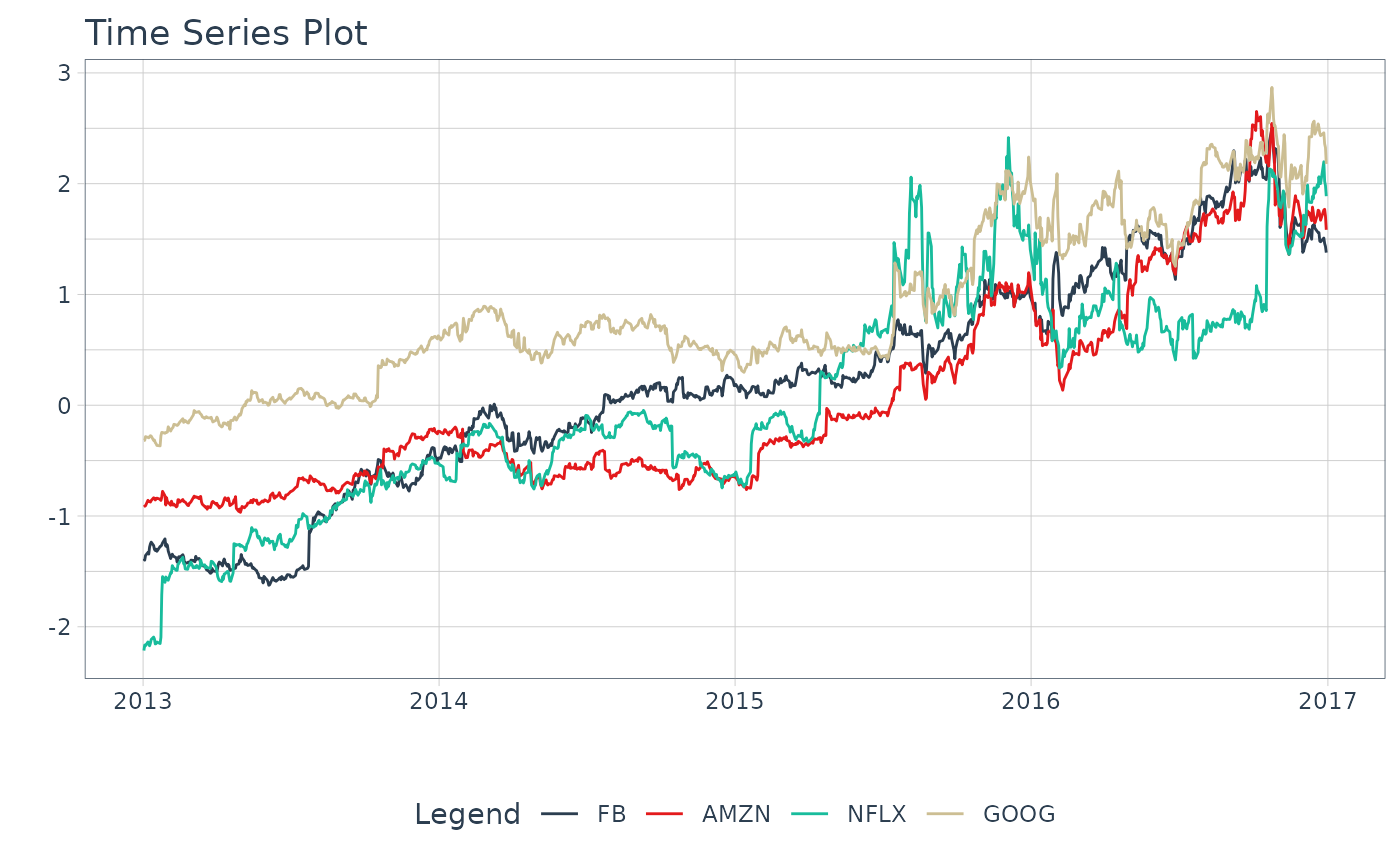step_log_interval creates a specification of a recipe step that will transform data using a Log-Inerval transformation. This function provides a recipes interface for the log_interval_vec() transformation function.

## Usage

step_log_interval(
recipe,
...,
limit_lower = "auto",
limit_upper = "auto",
offset = 0,
role = NA,
trained = FALSE,
limit_lower_trained = NULL,
limit_upper_trained = NULL,
skip = FALSE,
id = rand_id("log_interval")
)

# S3 method for step_log_interval
tidy(x, ...)

## Arguments

recipe

A recipe object. The step will be added to the sequence of operations for this recipe.

...

One or more selector functions to choose which variables are affected by the step. See selections() for more details. For the tidy method, these are not currently used.

limit_lower

A lower limit. Must be less than the minimum value. If set to "auto", selects zero.

limit_upper

An upper limit. Must be greater than the maximum value. If set to "auto", selects a value that is 10% greater than the maximum value.

offset

An offset to include in the log transformation. Useful when the data contains values less than or equal to zero.

role

Not used by this step since no new variables are created.

trained

A logical to indicate if the quantities for preprocessing have been estimated.

limit_lower_trained

A numeric vector of transformation values. This is NULL until computed by prep().

limit_upper_trained

A numeric vector of transformation values. This is NULL until computed by prep().

skip

A logical. Should the step be skipped when the recipe is baked by bake.recipe()? While all operations are baked when prep.recipe() is run, some operations may not be able to be conducted on new data (e.g. processing the outcome variable(s)). Care should be taken when using skip = TRUE as it may affect the computations for subsequent operations.

id

A character string that is unique to this step to identify it.

x

A step_log_interval object.

## Value

An updated version of recipe with the new step added to the sequence of existing steps (if any). For the tidy method, a tibble with columns terms (the selectors or variables selected) and value (the lambda estimate).

## Details

The step_log_interval() function is designed specifically to handle time series using methods implemented in the Forecast R Package.

Positive Data

If data includes values of zero, use offset to adjust the series to make the values positive.

Implementation

Refer to the log_interval_vec() function for the transformation implementation details.

Time Series Analysis:

• Engineered Features: step_timeseries_signature(), step_holiday_signature(), step_fourier()

• Diffs & Lags step_diff(), recipes::step_lag()

• Smoothing: step_slidify(), step_smooth()

• Variance Reduction: step_log_interval()

• Imputation: step_ts_impute(), step_ts_clean()

• Padding: step_ts_pad()

Transformations to reduce variance:

• recipes::step_log() - Log transformation

• recipes::step_sqrt() - Square-Root Power Transformation

Recipe Setup and Application:

• recipes::recipe()

• recipes::prep()

• recipes::bake()

## Examples

library(dplyr)
library(recipes)

FANG_wide <- FANG %>%
tidyr::pivot_wider(names_from = symbol, values_from = adjusted)

recipe_log_interval <- recipe(~ ., data = FANG_wide) %>%
step_log_interval(FB, AMZN, NFLX, GOOG, offset = 1) %>%
prep()
#> $FB #>  0 #> #>$FB
#>  145.318
#>
#> $AMZN #>  0 #> #>$AMZN
#>  904.973
#>
#> $NFLX #>  0 #> #>$NFLX
#>  143.7086
#>
#> $GOOG #>  0 #> #>$GOOG
#>  860.3125
#>

recipe_log_interval %>%
bake(FANG_wide) %>%
tidyr::pivot_longer(-date) %>%
plot_time_series(date, value, name, .smooth = FALSE, .interactive = FALSE)
#> $FB #>  0 #> #>$FB
#>  145.318
#>
#> $AMZN #>  0 #> #>$AMZN
#>  904.973
#>
#> $NFLX #>  0 #> #>$NFLX
#>  143.7086
#>
#> $GOOG #>  0 #> #>$GOOG
#>  860.3125
#>recipe_log_interval %>% tidy(1)
#> # A tibble: 4 × 5
#>   terms limit_lower limit_upper offset id
#>   <chr>       <dbl>       <dbl>  <dbl> <chr>
#> 1 FB              0        145.      1 log_interval_nUKOy
#> 2 AMZN            0        905.      1 log_interval_nUKOy
#> 3 NFLX            0        144.      1 log_interval_nUKOy
#> 4 GOOG            0        860.      1 log_interval_nUKOy